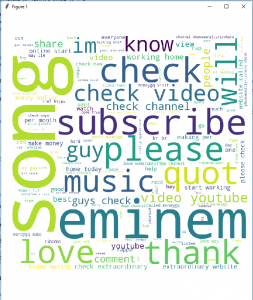# Generating Word Cloud in Python

• Difficulty Level : Medium
• Last Updated : 05 Jul, 2021

Word Cloud is a data visualization technique used for representing text data in which the size of each word indicates its frequency or importance. Significant textual data points can be highlighted using a word cloud. Word clouds are widely used for analyzing data from social network websites.

For generating word cloud in Python, modules needed are – matplotlib, pandas and wordcloud. To install these packages, run the following commands :

```pip install matplotlib
pip install pandas
pip install wordcloud```

The dataset used for generating word cloud is collected from UCI Machine Learning Repository. It consists of YouTube comments on videos of popular artists.

Below is the implementation :

## Python3

 `# Python program to generate WordCloud` `# importing all necessary modules``from` `wordcloud ``import` `WordCloud, STOPWORDS``import` `matplotlib.pyplot as plt``import` `pandas as pd` `# Reads 'Youtube04-Eminem.csv' file``df ``=` `pd.read_csv(r``"Youtube04-Eminem.csv"``, encoding ``=``"latin-1"``)` `comment_words ``=` `''``stopwords ``=` `set``(STOPWORDS)` `# iterate through the csv file``for` `val ``in` `df.CONTENT:``    ` `    ``# typecaste each val to string``    ``val ``=` `str``(val)` `    ``# split the value``    ``tokens ``=` `val.split()``    ` `    ``# Converts each token into lowercase``    ``for` `i ``in` `range``(``len``(tokens)):``        ``tokens[i] ``=` `tokens[i].lower()``    ` `    ``comment_words ``+``=` `" "``.join(tokens)``+``" "` `wordcloud ``=` `WordCloud(width ``=` `800``, height ``=` `800``,``                ``background_color ``=``'white'``,``                ``stopwords ``=` `stopwords,``                ``min_font_size ``=` `10``).generate(comment_words)` `# plot the WordCloud image                      ``plt.figure(figsize ``=` `(``8``, ``8``), facecolor ``=` `None``)``plt.imshow(wordcloud)``plt.axis(``"off"``)``plt.tight_layout(pad ``=` `0``)` `plt.show()`

Output :The above word cloud has been generated using Youtube04-Eminem.csv file in the dataset. One interesting task might be generating word clouds using other csv files available in the dataset.

1. Analyzing customer and employee feedback.
2. Identifying new SEO keywords to target.

Drawbacks of Word Clouds :

1. Word Clouds are not perfect for every situation.
2. Data should be optimized for context.

Reference : https://en.wikipedia.org/wiki/Tag_cloud

My Personal Notes arrow_drop_up